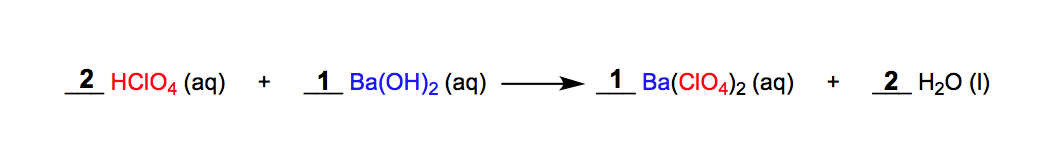Clutch Prep is now a part of Pearson
Ch.5 Classification & Balancing of Chemical ReactionsWorksheetSee all chapters

# Molecular Equations

See all sections
Sections
Chemical Reaction: Chemical Change
Law of Conservation of Mass
Balancing Chemical Equations (Simplified)
Solubility Rules
Molecular Equations
Types of Chemical Reactions
Complete Ionic Equations
Calculate Oxidation Numbers
Redox Reactions
Spontaneous Redox Reactions
Balancing Redox Reactions: Acidic Solutions
Balancing Redox Reactions: Basic Solutions
Balancing Redox Reactions (Simplified)
Galvanic Cell (Simplified)

Molecular Equations show reacting molecules involved in a chemical reaction.

###### Examining Molecular Equations

Concept #1: Introduction to Molecular Equations

In a typical molecular equation, two reactants dissociate and reorganize to form new productsExample #1: Which of the following is a precipitation reaction?

Example #2: Predict whether a chemical reaction occurs and write the balanced molecular equation.

A reaction occurs only if solid, gas or liquid water is formed. If both products are aqueous, NO REACTION has occurred.

Practice: Predict whether a chemical reaction occurs and write the balanced molecular equation.

Ag2SO4 (aq) + KCl (aq) →

Practice: Predict whether a chemical reaction occurs and write the balanced molecular equation.

MgBr2 (aq) +  NaC2H3O2 (aq) →

Practice: Determine the balanced equation for the neutralization equation

Ca(OH)2 (aq) + HCN (aq) →